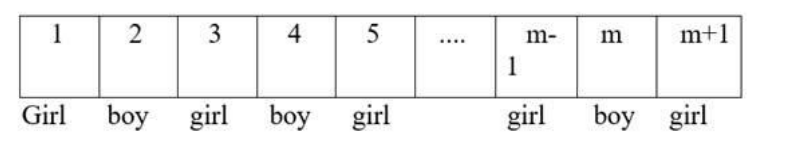# Find the number of ways in which m boys and n girls may be arranged in a

Question:

Find the number of ways in which m boys and n girls may be arranged in a row so that no two of the girls are together; it is given that m > n.

Solution:

In this question, n girls are to be seated alternatively between m boys.

There are m+1 spaces in which girls can be arranged.The number of ways of arranging $n$ girls is $P(m+1, n)=\frac{(m+1) !}{(m-n+1) !}$ ways.

Formula:

Number of permutations of $n$ distinct objects among $r$ different places, where repetition is not allowed, is

$P(n, r)=n ! /(n-r) !$

Therefore, permutation of n different objects in m+1 places is

$P(m+1, n)=\frac{(m+1) !}{(m+1-n) !}$

$=\frac{(m+1) !}{(m-n+1) !}$

The arrangement of m boys can be done in P(m, m) ways.

Formula:

Number of permutations of n distinct objects among r different places, where repetition is not allowed, is

$P(n, r)=n ! /(n-r) !$

Therefore, a permutation of m different objects in m places is

$P(m, m)=\frac{\frac{m !}{(m-m) !}}{(m-0 !}=\frac{m !}{m !}$

Therefore the total number of arrangements is $\frac{(\mathrm{m}+1) !}{(\mathrm{m}-\mathrm{n}+1) !} \times \mathrm{m} !$